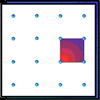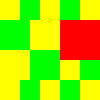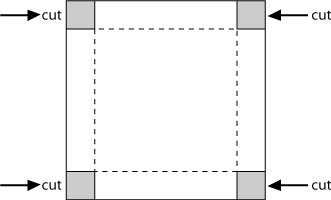#### You may also like### Geoboards

This practical challenge invites you to investigate the different squares you can make on a square geoboard or pegboard.### Tiles on a Patio

How many ways can you find of tiling the square patio, using square tiles of different sizes?### Pebbles

Place four pebbles on the sand in the form of a square. Keep adding as few pebbles as necessary to double the area. How many extra pebbles are added each time?

# Making Boxes

##### Age 7 to 11Challenge Level

In this problem you start with some sheets of squared paper measuring $15\times 15$ and use them to make little boxes without lids.

You do this by cutting out squares at the corners and then folding up the sides. (The folds are indicated by the dotted lines in the diagram.)Begin by cutting one square out of each corner. Fold up the sides. What is the size of the base? How high are the sides? So what is its volume?

Now cut a $2 \times 2$ square out of each corner and fold up the sides.
Does it look as if it holds more than the first box, less than the first box or just the same amount?
What is the size of the base now? How high are the sides now? So what is its volume?

Now cut a $3 \times 3$ square out of each corner and fold up the sides.
Does it look as if it holds more than the other boxes, less than the other boxes or just the same amount?
What is the size of the base now? How high is it now? So what is its volume?

If you keep on doing this, taking larger and larger squares from the corners, which box will have the largest volume?